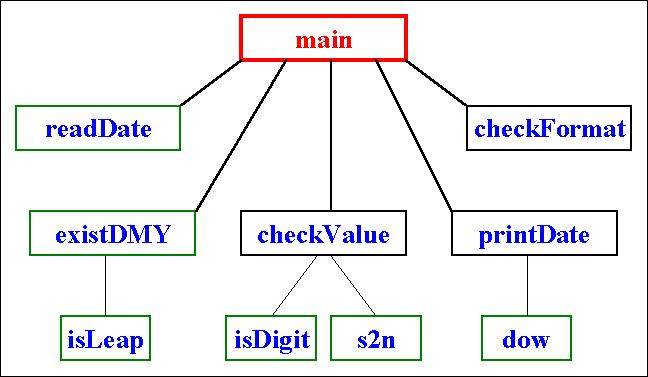# LAB 9

## Objectives

• Structured Problem-Solving
• Functions and Subroutines

## Date Validation & Conversion

Given a date like 20/2/2002, we like to convert it to: Wed. Feb 20, 2002. The process involves validating the input to ensure it has the proper format and then extracting from it the day, month, and year as strings. Assuming the extracted fields are indeed numeric, we convert them to integers and then verify that the date exists; e.g. it is not the 31st of September or the 29th of February in a non-leap year. Once properly validated, we employ the Zeller algorithm to find the day of the week (dow) and then output the result looking up the month name and the day name.

Here is how the sought system should behave:

` `
` Enter the date ...`
`test`
` Invalid format!`
` Enter the date ...`
`test/`
` Invalid format!`
` Enter the date ...`
`t/mm/yyyy`
` Non-numeric values!`
` Enter the date ...`
`12/45/98`
` None existent date!`
` Enter the date ...`
`10/2/1983`
` Thu. Feb 10, 1983`

It would be a mistake to attempt this using the un-structured approach. This would yield messy code that is hard to debug and understand. Instead, we break the problem into pieces as shown in the following structure chart:Here is the full main program:

` `
`program LongDate`
`implicit none`
`character*10 date, day, month, year, readDate`
`integer*2 d, m, y`
`logical*1 ok, existDMY`
` `
`do while (.true.)`
`   ok = .true.`
`   date = readDate()`
`   call checkFormat(date, day, month, year, ok)`
`   if (.not. ok) then`
`      print*, "Invalid format!"`
`   else`
`      call checkValue(day, month, year, d, m, y, ok)`
`      if (.not. ok) then`
`         print*, "Non-numeric values!"`
`      else`
`         if (existDMY(d, m, y)) then`
`            call printDate(d, m, y)`
`         else`
`            print*, "None existent date!"`
`            ok = .false.`
`         end if`
`      end if`
`   end if`
`   if (ok) then`
`      exit`
`   end if`
`end do`
`end`

The job description of each subprogram is as follows:

• `readDate() `
prompt for a date, read it as a string, return it to the caller.
• `checkFormat `
verify that the date string has two slashes and extract and return the three substrings delimited by these slashes. Return false if the extraction was not possible. Note that this sub does not care about the content of the three strings, only that they exist; e.g. they can contain non-digits but it still returns `true`.
• `checkValue `
verify that the three passed strings are indeed made up exclusively of digits and return them as integers. If not, return `false`.
• `existDMY `
verify that the passed integers do indeed represent a date that exists (month between 1 and 12 and day between 1 and 28 or 29 or 30 or 31 depending on the month and year). If not, return `false`.
• `isLeap `
verify that the passed year is a leap year. If not, return `false`.
• `isDigit `
verify that the passed string is made up of digits (plus possible trailing spaces). If any character in the string is not a digit nor a space, return `false` else return `true`.
• `s2n `
convert the passed string, assuming it is made up exclusively of digits (plus possible trailing spaces) to the corresponding integer value.
• `printDate `
Output the date by translating Zeller's dow code into Sun or Mon, or ... Sat and outputting this day name followed by a dot. The month number is also translated into a month name (Jan Feb Mar ... Dec). The final format is: ddd. mmm dd, yyyy (where ddd is the day name and mmm is the month name).
• `dow `
determine the day of the week and return it as 0 for Sunday, 1 for Monday, ... and 6 for Saturday. This sub uses an algorithm due to Zeller, as follows:
`·                  `
`·                            mm = m`
`·                            yy = y`
`·                            if (m .le. 2) then`
`·                               mm = m + 12`
`·                               yy = y - 1`
`·                            end if`
`·                            tmp1 = 26*(mm+1) / 10`
`·                            tmp2 = 125*yy / 100`
`·                            tmp3 = d + tmp1 + tmp2 - yy / 100 + yy / 400 - 1`
`      dow = mod(tmp3, 7)`

It would also be a mistake if these subprograms are written in full, put together, and then tested. Instead, write only one of them, any one, and then write a small main program that uses it. Such a main program is known as a driver or a test harness and it enables you to test each piece before building the whole system. Only when all pieces are tested individually, and only then, would you put them together and test. You can put everything in one giant source file but it is recommended that those subprograms that can be useful for other applications (such as `s2n` or `isLeap`) be placed in separate files and compiled using `f2lib`.

### Deliverables

1. Printed program
2. A log showing the program's input and output for at least 10 cases so that all possible outcomes (errors or valid results) are demonstrated.

Fall2002/HR